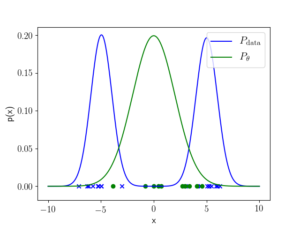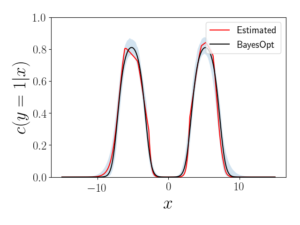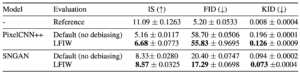# Are all samples created equal?: Boosting generative models via importance weighting

This post has been republished via RSS; it originally appeared at: Microsoft Research.By Aditya Grover, PhD Candidate, Stanford University

There is a growing interest in the use of deep generative models for sampling high-dimensional data; examples include high-resolution natural images, long-form text generation, designing pharmaceutical drugs, and creating new materials at the molecular level. Training these models is, however, an arduous task. Even state-of-the-art models have noticeable deficiencies in some of the generated samples: image models of faces have artifacts in the hair textures and makeup, text models often require repeated attempts at generating coherent completions of sentences or paragraphs, and other deficiencies. In these cases, cherry-picking good samples is not a scalable alternative.

In a paper presented last month at the thirty-third Conference on Neural Information Processing Systems (NeurIPS 2019), called “Bias Correction of Learned Generative Models using Likelihood-Free Importance Weighting,” our team of researchers at Microsoft and Stanford University propose a scalable algorithmic approach to characterize and mitigate the imperfections of generative models. Our technique consistently improves sample quality metrics for state-of-the-art generative models while also benefiting downstream use cases of generative models for data augmentation and off-policy policy evaluation.

### Importance weighting induces an energy-based generative model

Let’s say we are given a generative model p_theta (such as any variational autoencoder, generative adversarial network, or other model) that has been trained to learn a data distribution p_data. Our goal is to characterize and mitigate the imperfections of this model. To do this, we consider any non-negative weighting function w_phi and combine it with our base model to induce an energy-based model with density:

p_{theta, phi}(x) \propto p_theta(x) w_phi(x)

The above model is an instantiation of a product-of-experts (PoE) model as it boosts a base (normalized) model p_theta multiplicatively using a weighting function w_phi.

#### What’s the ideal weighting function?

If the weighting function corresponds to the ratio of data density to the model density (that is, w_phi(x)=p_data(x)/p_theta(x) for all x), then the energy-based model recovers the data distribution (that is, p_{theta, phi}=p_data). In such a scenario, w_phi(x) is the importance weighting function for debiasing expectations under the data distribution (also known as the “target” in Monte Carlo terminology) given access to only the model distribution (or “proposal”).

#### How do we estimate the importance weights?

In order to compute the density ratio, the data density (the numerator) is unavailable and model density (the denominator) is often intractable in practice in the case of variational autoencoders, generative adversarial networks, and many other generative models. To get rid of this shortcoming, we use probabilistic binary classifiers to estimate the density ratio—in particular, the estimator is the odds ratio of a classifier trained to distinguish data samples from the generated samples. If the classifier is Bayes optimal, the importance weights are exact. Appealingly, this procedure is “likelihood-free” as it does not involve knowing the model or the data density. A toy example is shown below.Figure 1: univariate Gaussian (green) is fit to a mixture of two Gaussians (blue).Figure 2: estimated (red) and Bayes optimal (black, BayesOpt) class probabilities (with 95% confidence intervals based on 1,000 bootstraps) for a classifier trained to distinguish 1,000 true data and generated data samples.

#### How do we sample from the induced model?

Exact sampling from the induced energy-based model is computationally intractable. However, we can leverage a resampling technique, called Sampling Importance Resampling (SIR), to sample from an approximation to the energy-based model. Given a positive integer parameter k, SIR prescribes a 3-step procedure:

(1) Generate k independent samples from the base model p_theta.
(2) Estimate importance weights for the k samples.
(3) Resample from these k samples in proportion to the importance weights.

In the limit of k going to infinity, we will exactly sample from the energy-based model. Therefore, for any finite budget k, we can trade accuracy for computational efficiency or vice versa.

### Application use cases

We evaluate several standard sample quality metrics on the CIFAR-10 dataset for state-of-the-art likelihood-based and likelihood-free models with and without our proposed debiasing technique (denoted as likelihood-free importance weighting or LFIW). The weights here were estimated using a neural network performing binary classification. Our technique consistently improves on these metrics, suggesting reduced bias in evaluation.Table 1: Goodness-of-fit evaluation on CIFAR-10 dataset for PixelCNN++ and SNGAN. Standard errors computed over 10 runs. Higher Inception Scores (IS) are better. Lower Frechet Inception Distance (FID) and Kernel Inception Distance (KID) scores are better.

Besides improved sample-quality metrics, we show the benefits of our approach for:

• data augmentation on Omniglot datasets using generative adversarial networks: weighting the contributions of the good and bad generations in the training loss improves classification accuracy.
• model-based off-policy policy evaluation on MuJoCo environments: weighting the contributions of simulated trajectories under the dynamics model (learned using off-policy data) leads to better estimates of the policy of interest.

In summary, we present a simple, yet highly effective technique based on importance weighting to correct for the imperfections of generative models by inducing a boosted energy-based model. While the proposed technique can correct for the model bias, the datasets used for training could also be biased (as is the case when the training dataset is scraped from Internet sites, such as Reddit), and our follow-up work uses similar techniques to mitigate dataset bias for achieving fairness in generative modeling.

The post Are all samples created equal?: Boosting generative models via importance weighting appeared first on Microsoft Research.

This site uses Akismet to reduce spam. Learn how your comment data is processed.